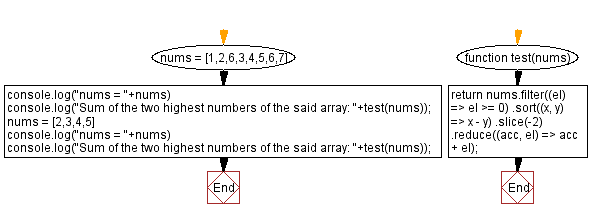# JavaScript: Sum of the Two highest Numbers

## JavaScript Math: Exercise-84 with Solution

Write a JavaScript program to calculate the sum of the two highest positive numbers in a given array.

Test Data:
([1,2,6,3,4,5,6,7]) ->13
([2,3,4,5]) -> 9

Sample Solution:

HTML Code:

``````<!DOCTYPE html>
<html>
<meta charset="utf-8">
<title>JavaScript program to Sum of the Two highest Numbers</title>
<body>

</body>
</html>
```
```

JavaScript Code:

``````function test(nums) {
return nums.filter((el) => el >= 0)
.sort((x, y) => x - y)
.slice(-2)
.reduce((acc, el) => acc + el);
}
nums = [1,2,6,3,4,5,6,7]
console.log("nums = "+nums)
console.log("Sum of the two highest numbers of the said array: "+test(nums));
nums = [2,3,4,5]
console.log("nums = "+nums)
console.log("Sum of the two highest numbers of the said array: "+test(nums));
```
```

Sample Output:

```nums = 1,2,6,3,4,5,6,7
Sum of the two highest numbers of the said array: 13
nums = 2,3,4,5
Sum of the two highest numbers of the said array: 9
```

Flowchart:Live Demo:

See the Pen javascript-math-exercise-84 by w3resource (@w3resource) on CodePen.

Improve this sample solution and post your code through Disqus

What is the difficulty level of this exercise?

Test your Programming skills with w3resource's quiz.

﻿